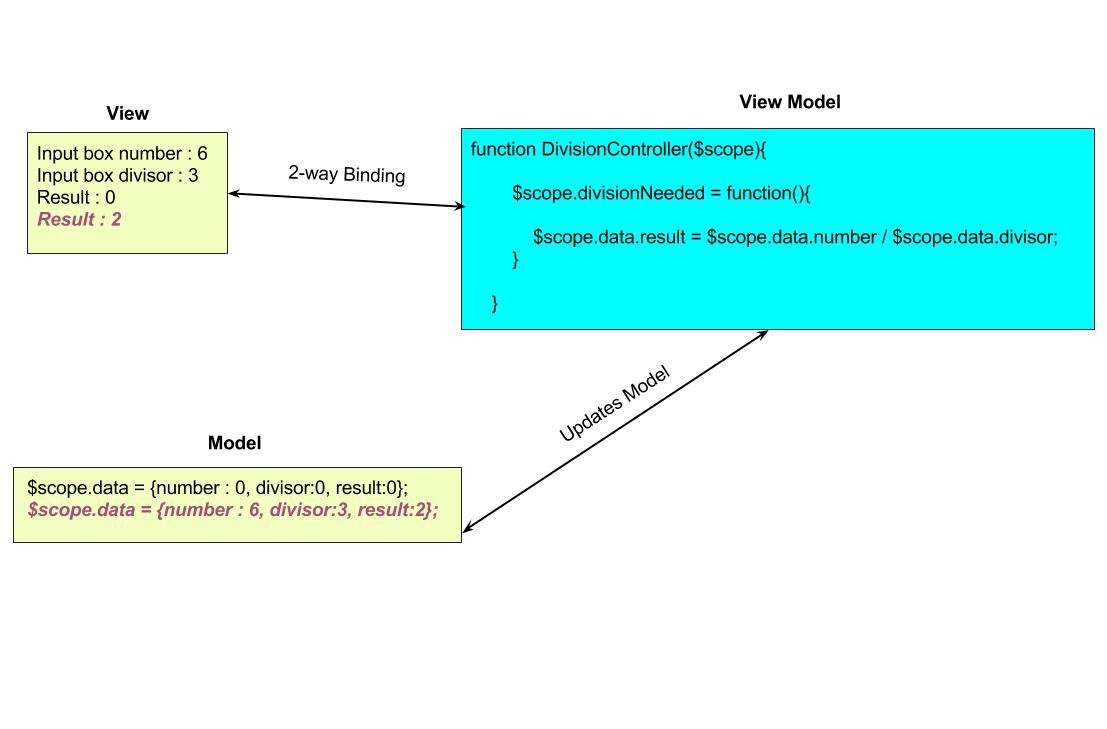# MVC and MVVM with AngularJS

Design Pattern is nothing but a maintainable and reusable way of writing code which can be applied to commonly occurring problems. There are numerous design patterns such as MVC, MVVM, DI etc. It depends upon the problem that which design pattern needs to be followed.

This blog emphasizes on MVC and MVVM design patterns with reference to AngularJS.

Let's get started:

MVC Design Pattern

To start with, MVC design pattern is not specific to AngularJS, you must have seen/implemented this pattern in many other programming languages.

MVC design pattern can be seen in AngularJS, but before getting into that let's see what all does MVC design pattern includes:

1. Model: Model is nothing but data.
2. View: View represents this data.
3. Controller: Controller mediates between the two.

Let's see the code below:

```<!DOCTYPE html>
<html ng-app>
<title>MVC</title>
<script type="text/javascript" src="angular.min.js"></script>
<body ng-controller="TextController">
<p>{{sampleText}}</p>
</body>
<script>
function TextController(\$scope) {
\$scope.sampleText = 'This is a demo!';
}
</script>
</html>```

In the above snippet, our text is represented by "sampleText" variable. This is our model. Controller is updating the view by displaying the data on the view by replacing "{{sampleText}}" with sampleText variable value. It is the controller that is managing the relationship between our model and the view which is the HTML.

In MVC if we make any change in the the view it doesn't gets updated in model. But in AngularJS, we have heard that there is something called 2-way binding and this 2-way binding enables MVVM design pattern. MVVM basically includes 3 things:

1. Model
2. View
3. View Model

Controller is actually replaced by View Model in MMVM design pattern. View Model is nothing but a JavaScript function which is again like a controller and is responsible for maintaining relationship between view and model, but the difference here is, if we update anything in view, it gets updated in model, change anything in model, it shows up in view, which is what we call 2-way binding.

Let's see the example given below:

```<!DOCTYPE html>
<html ng-app>
<title>Number Divisor</title>
<script type="text/javascript" src="angular.min.js"></script>
<body>
<form ng-controller="DivisionController">
<label>Number :</label> <input name="number" ng-change="divisionNeeded()" ng-model="data.number">
<label>Number entered by User :</label> {{data.number}} <br>
<label>Divisor :</label> <input name="divisor" ng-change="divisionNeeded()" ng-model="data.divisor">
<label>Number entered by User :</label> {{data.divisor}} <br>
<label>Result :</label> {{data.result}}
</form>
</body>
<script>
function DivisionController(\$scope) {
\$scope.data = {number: 0, divisor: 0, result: 0};
\$scope.divisionNeeded = function () {
\$scope.data.result = \$scope.data.number / \$scope.data.divisor;
}
}
</script>
</html>```

The above code contains 2 input boxes which takes number and a divisor to divide that number. The result of the division is shown in front of the label 'Result'.

Also, whatever is input in those two input boxes is displayed in front of the input box itself. This code shows the MVVM design pattern in JavaScript very clearly, here you see how:The above diagram segregates the code into Model, View and View Model.

I am first starting with view. We know that view is our HTML,so we update it by entering '6' in the number text box and '3' in the divisor text box. As soon as any change is made in the view, View Model gets to know about it, and these values are then updated into the Model. Apart from this, the main work is that is happening in View Model is the division, number is getting divided by the divisor and is the result is being assigned to 'data.result' variable. Here, since 'data.result' is our model, and as there is change here from it's initial value of '0' to '2' now, view gets updated and the result of the division is shown on the HTML.

This is why we say AngularJS follows MVVM design pattern.